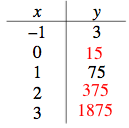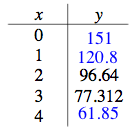### Home > CCA > Chapter Ch7 > Lesson 7.1.3 > Problem7-36

7-36.

Each table below represents an exponential function in $y=ab^x$ form. Copy and complete each table on your paper and find a corresponding equation. Homework Help ✎

1.  x y –1 3 0 1 75 2 3

• What is the multiplier?

• y = 15 · 5x

•1.  x y 0 1 2 96.64 3 77.312 4